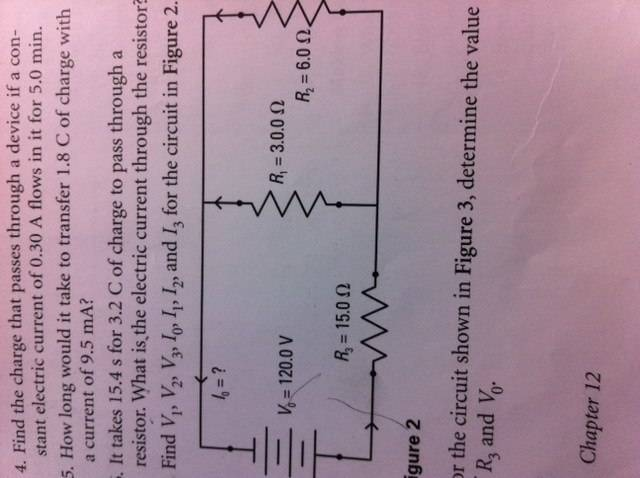# Electricity - Solving unknown variables for mixed circuits

• Engineering
• anonymous12

## Homework Statement

7. Find V1, V2, V3, I0, I1, I2, and I3 for the circuit in Figure 2.V0 = 120V
R3=15 ohms
R1=3 ohms
R2 = 6 ohms

Kirchoff's law
Ohm's law

## The Attempt at a Solution

R0 = R1 + R3
= 3 + 15
=18 ohms

I0=V0/R0
=120/128
=6.66A

I1=I3=I0
I1=I3=6.66A

V3=R0 X I3
= 18 X 6.66
=99.9V

V1=V0 - V3
=120-99.9
=20.1V

V2=V1
V1=20.1V

I2 = V2/R2
= 20.1/6
=3.35A

This is what I did but when I look at the back of the textbook it gives me different answers.

Last edited: